# Trung tâm đào tạo thiết kế vi mạch Semicon

• Đăng ký
 Name: * Tên đăng nhập: * Email: * Mật khẩu: * Verify Password: * Fields marked with an asterisk (*) are required.# Thế giới ASIC

## User Defined Primitives Part-IIILevel Sensitive Sequential UDP
Level-sensitive sequential behavior is represented in the same way as combinational behavior, except that the output is declared to be of type reg, and there is an additional field in each table entry. This new field represents the current state of the UDP.

Lần cập nhật cuối ( Thứ bảy, 04 Tháng 9 2021 13:42 ) Đọc thêm...

## User Defined Primitives Part-IICombinational UDPs

In combinational UDPs, the output is determined as a function of the current input. Whenever an input changes value, the UDP is evaluated and one of the state table rows is matched.

Lần cập nhật cuối ( Thứ bảy, 04 Tháng 9 2021 13:35 ) Đọc thêm...

## User Defined Primitives Part-IIntroduction

Verilog has built-in primitives like gates, transmission gates, and switches. This is a rather small number of primitives; if we need more complex primitives, then Verilog provides UDP,

Lần cập nhật cuối ( Thứ bảy, 04 Tháng 9 2021 13:21 ) Đọc thêm...

## My first program in Verilog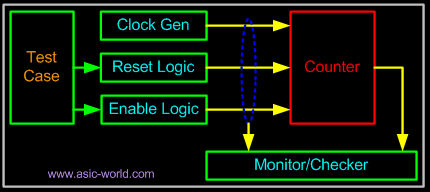Introduction

If you refer to any book on programming languages, it starts with an "Hello World" program; once you have written it, you can be sure that you can do something in that language

Lần cập nhật cuối ( Thứ ba, 31 Tháng 8 2021 15:34 ) Đọc thêm...

## Art of Writing TestBenches Part - II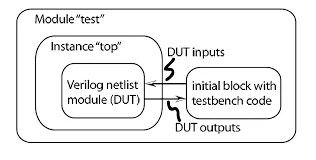Writing a TestBench

First step of any testbench creation is building a dummy template which basically declares inputs to DUT as reg and outputs from DUT as wire, then instantiates the DUT as shown in the code below.

Lần cập nhật cuối ( Thứ ba, 31 Tháng 8 2021 15:22 ) Đọc thêm...

## Art of Writing TestBenches Part - IIntroduction
Writing a testbench is as complex as writing the RTL code itself. These days ASICs are getting more and more complex and thus verifying these complex ASIC has become a challenge. Typically 60-70% of time needed for any ASIC is spent on verification/validation/testing.

Lần cập nhật cuối ( Thứ ba, 31 Tháng 8 2021 15:16 ) Đọc thêm...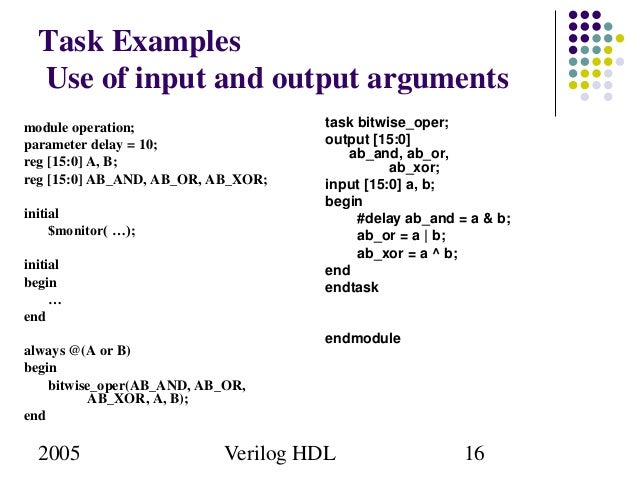Introduction

There are tasks and functions that are used to generate input and output during simulation. Their names begin with a dollar sign (\$). The synthesis tools parse and ignore system functions, and hence can be included even in synthesizable models.

Lần cập nhật cuối ( Chủ nhật, 29 Tháng 8 2021 14:12 ) Đọc thêm...

## Compiler Directives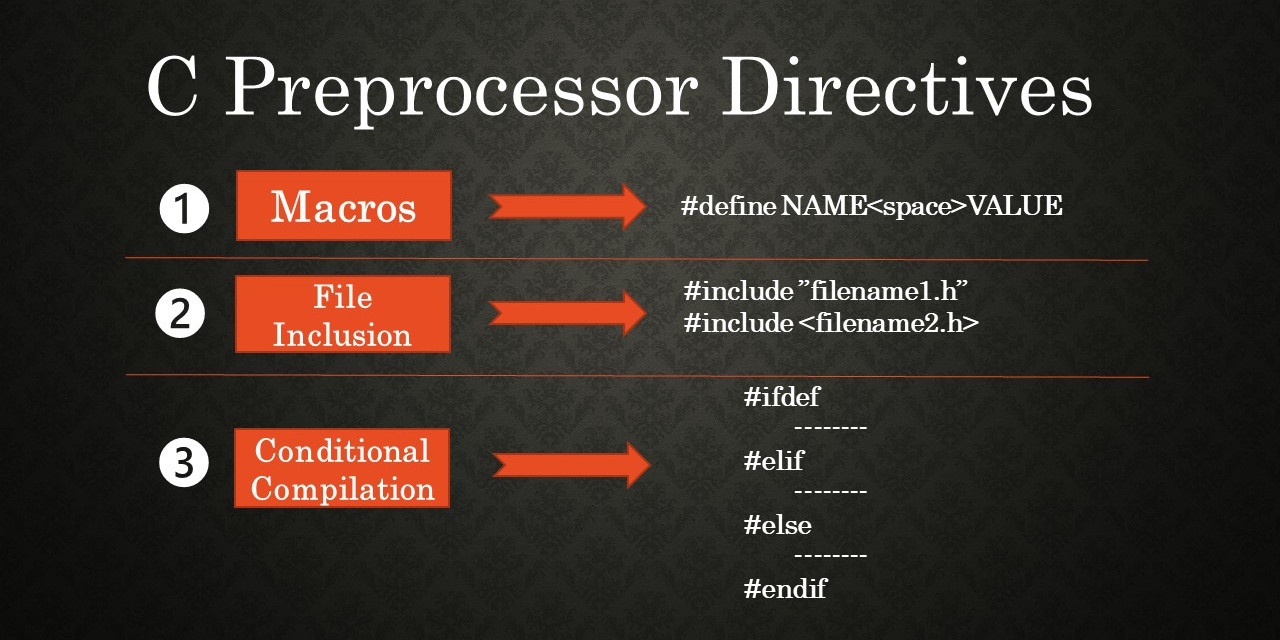Introduction

A compiler directive may be used to control the compilation of a Verilog description. The grave accent mark, `, denotes a compiler directive.

Lần cập nhật cuối ( Chủ nhật, 29 Tháng 8 2021 14:01 ) Đọc thêm...

## Modeling Memories And FSM Part - II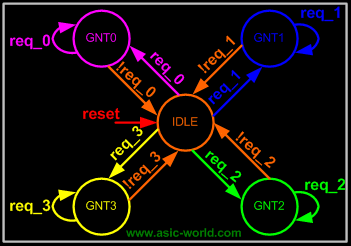Introduction to FSMState machines or FSM are the heart of any digital design; of course a counter is a simple form of FSM. When I was learning Verilog, I used to wonder "How do I code FSM in Verilog"
and "What is the best way to code it". I will try to answer the first part of the question below and second part of the question can be found in the tidbits section.

State machine Types

There are two types of state machines as classified by the types of outputs generated from each. The first is the Moore State Machine where the outputs are only a function of the present state, the second is the Mealy State Machine where one or more of the outputs are a function of the present state and one or more of the inputs.

 Mealy Model

Moore Model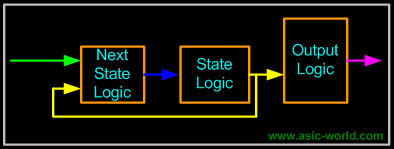State machines can also be classified according to the state encoding used. Encoding style is also a critical factor which decides speed and gate complexity of the FSM. Binary, gray, one hot, one cold, and almost one hot are the different types of encoding styles used in coding FSM states.

Modeling State machines.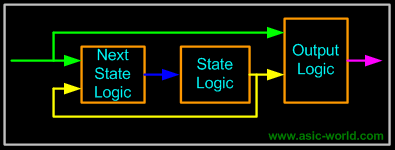One thing that need to be kept in mind when coding FSM is that combinational logic and sequence logic should be in two different always blocks. In the above two figures, next state logic is always the combinational logic. State Registers and Output logic are sequential logic. It is very important that any asynchronous signal to the next state logic be synchronized before being fed to the FSM. Always try to keep FSM in a separate Verilog file.
Using constants declaration like parameter or `define to define states of the FSM makes code more readable and easy to manage.
 Example - Arbiter

We will be using the arbiter FSM to study FSM coding styles in Verilog

Verilog Code
 FSM code should have three sections:
• Encoding style.
• Combinational part.
• Sequential part.
 Encoding Style

There are many encoding styles around, some of which are:

• Binary Encoding
• One Hot Encoding
• One Cold Encoding
• Almost One Hot Encoding
• Almost One Cold Encoding
• Gray Encoding

Of all the above types we normally use one hot and binary encoding.

 One Hot Encoding 1 parameter  [4:0]  IDLE  = 5'b0_0001;  2 parameter  [4:0]  GNT0  = 5'b0_0010;  3 parameter  [4:0]  GNT1  = 5'b0_0100;  4 parameter  [4:0]  GNT2  = 5'b0_1000;  5 parameter  [4:0]  GNT3  = 5'b1_0000; Binary Encoding 1 parameter  [2:0]  IDLE  = 3'b000;  2 parameter  [2:0]  GNT0  = 3'b001;  3 parameter  [2:0]  GNT1  = 3'b010;  4 parameter  [2:0]  GNT2  = 3'b011;  5 parameter  [2:0]  GNT3  = 3'b100; Gray Encoding 1 parameter  [2:0]  IDLE  = 3'b000;  2 parameter  [2:0]  GNT0  = 3'b001;  3 parameter  [2:0]  GNT1  = 3'b011;  4 parameter  [2:0]  GNT2  = 3'b010;  5 parameter  [2:0]  GNT3  = 3'b110;

Bạn Có Đam Mê Với Vi Mạch hay Nhúng      -     Bạn Muốn Trau Dồi Thêm Kĩ Năng

Mong Muốn Có Thêm Cơ Hội Trong Công Việc

Và Trở Thành Một Người Có Giá Trị Hơn

# Hãy Để Chúng Tôi Hỗ Trợ Cho Bạn. SEMICONLần cập nhật cuối ( Chủ nhật, 29 Tháng 8 2021 13:55 )

## Modeling Memories And FSM Part - IMemory Modeling To help modeling of memory, Verilog provides support for two dimensions arrays. Behavioral models of memories are modeled by declaring an array of register variables; any word in the array may be accessed using an index into the array. A temporary variable is required to access a discrete bit within the array.

Lần cập nhật cuối ( Thứ bảy, 28 Tháng 8 2021 15:25 ) Đọc thêm...

## Verilog Quick Reference part 2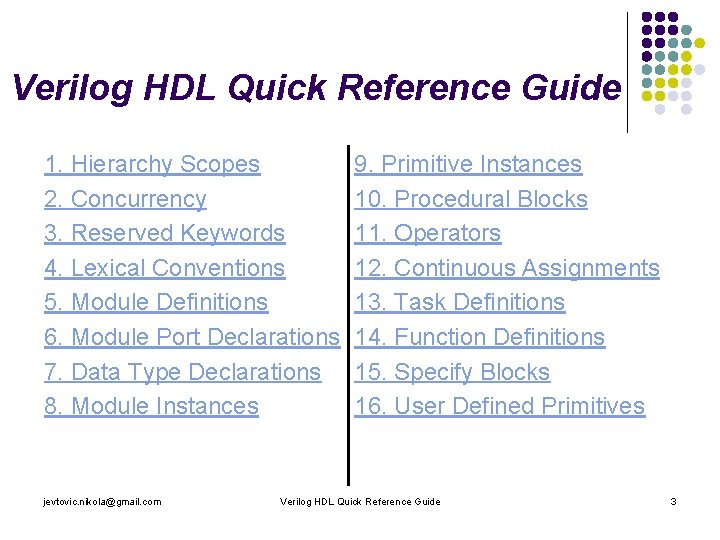SYSTEM TASKSbut mentioned in the informative appendix.

INPUT
Lần cập nhật cuối ( Thứ bảy, 28 Tháng 8 2021 14:58 ) Đọc thêm...

## Verilog Quick Reference part 1This is still in very early stage, need time to add more on this.

MODULE

module MODID[({PORTID,})];

Lần cập nhật cuối ( Thứ bảy, 28 Tháng 8 2021 14:51 ) Đọc thêm...

## Verilog Synthesis Tutorial Part-IIILogic Circuit Modeling

From what we have learnt in digital design, we know that there could be only two types of digital circuits. One is combinational circuits and the second is sequential circuits. There are very few rules that need to be followed to get good synthesis output and avoid surprises.

Lần cập nhật cuối ( Thứ năm, 26 Tháng 8 2021 20:27 ) Đọc thêm...

## Verilog Synthesis Tutorial Part-IIConstructs Not Supported in Synthesis

Lần cập nhật cuối ( Thứ năm, 26 Tháng 8 2021 20:17 ) Đọc thêm...

## Verilog Synthesis Tutorial Part-I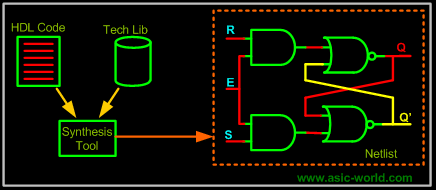What is logic synthesis ?

Logic synthesis is the process of converting a high-level description of design into an optimized gate-level representation. Logic synthesis uses a standard cell library which have simple cells, such as basic logic gates like and,

Lần cập nhật cuối ( Thứ năm, 26 Tháng 8 2021 19:34 ) Đọc thêm...

## Assertions In Verilog Part - IIIAssertion with OVL

Now that we have seen the code of FIFO and the testbench, let's see the example of using OVL to build assertions for the FIFO. To use OVL, we need to first install the OVL package.

Lần cập nhật cuối ( Chủ nhật, 22 Tháng 8 2021 21:20 ) Đọc thêm...

Các bài viết khác...
Trang 5 của 109